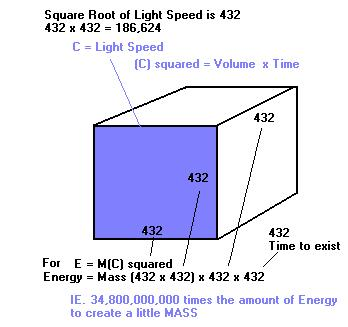432 - Key of 'Star of David'
Part FIVE
Speed of Light and 432

Einstein's famous equation of energy and matter was written  E=mc2  In other words,
there is a connection between energy (spirit) and matter via light speed squared as c =
light speed (SEE
Flesh and Spirit Equation) Why because matter came from energy or
SPIRIT. The
Lord was the CREATOR from the Invisable Spirit World.

From previous article at
Light Speeed proves God's Design

m = meter = 1/10,000,000 of the Earth's Circumference from Pole to Equator
M= megameter or 10,000,000 meters
c  = speed of light = 299,792,000 m/sec
d = s times t (distance equals speed multiplied by time)
A.U. = Distance from Earth to Sun
***************************************************
m = c(sec)/299,792,000
Therefore m= 1/10,000,000 of Earth's 1/4 Circumference = c(sec)/299,792,000

Do you see the relationship between the Earth and the Speed of Light and then when you add in the equation of the
Parthenon and
the Speed of Light , and the equation from the LIX NUMBER at http://www.klatu.com/lix/index.html
you get ....

c = phi (M) (600')/seconds
c = (A.U.)/500seconds = 4.9' x M (10,000) = phi ' x (M) (100,000)

(Notice how 500 seconds relates exactly to the Time for light speed to reach Earth -
Tabernacle of the Sun -
as well as the height of Giza and the
Cydonia Mound)

Therefore Light Speed and Phi are intimately related and that with the Distance between the Earth and the Sun. Because "Light
Speed is measured in Earth seconds and the mean distance of the Earth from the Sun is a function of the Golden Section and the
number 33". SEE
Absolute 33 and  Speed of Light, 33 and Phi connections

Following from .....

This leads to one of the most profound instances of the occurrence of the number 432. In the equation, E= energy, m = matter, and c
= the universal constant speed of light. The speed of light is generally accepted to be 186,291 miles per second in a vacuum.
However, the square of the number 432 is 186,624. If the universal constant speed of light were in fact 186,624, then the number
432 would be the square root of the speed of light .....

Square of 432.
Comment - Presume for a moment that you do not know the speed of light, but are aware of the equation E=mc2. An intuitive
scientist who substituted the number 432 for "c" in the equation would be very close, if not precise, in the determination of this
universal constant, that is, E=m(432)4. This illustrates the important concept of "reverse engineering" to find unknown quantities
and constants by use of the cosmic number 432 and its multiples and divisors.

Consequently if 432 is a distance of a side of a cube
(or string distance that vibrates at a certain hertz) then
a cube face would be equal to 432 x 432, which equals
186,642, close enough to be light speed because of the
anomales of light speed. SEE hyper LINK below.

Therefore it seems we can picture light speed as an,
area of 432. (SEE graphics to the right coloured in blue)

Yet to bring light speed (ENERGY) into MASS,
light speed has to be be multiplied by itself...as E = M(C) 2

Hence visualizing this in 3 Dimensions, gives us
a Cube with
432 sides. And if we give it time to exist as
its fourth dimension area of light speed could make a
cube and to make it exist in time it makes it 4 dimensional
with another factor of
432.

Consequently it takes a HUGE, HIGE amount of
organized ENERGY or Spirit or Light to produce just
a small amount of matter, and that is another reason why
there has to be a Creator of Almighty, un-exhaustable
Energy to be able to creat mass, us and the Universe.

And the major  archetype of
432, and its corresponding
vibrations and octaves and harmonics are KEY to being in
tune with Him and His Creation. Don't you agree ?

Still can't catch it, then consider the speed of light in a nanosecond, a billionth of a second ... it goes about about one foot. This
because new research shows that light speed can be altered, at different temperatures and is not an absolute constant.

Research News HERE ..... http://www.washingtonpost.com/wp-dyn/content/article/2007/01/18/AR2007011801683.html

This is why the Creator could create beyond the speed of light in the Beginning. For when He placed the stars seemingly light years
away in distance, they weren't because His Spirit is instantaneous everywhere

Then we can assume that light can travel exactly one foot per billionth of a second. A foot representing a human foot length, a span
..a human hand span, a cubit, three spans or one and a half feet, or length from our finger tips to elbow, means light speed itself is
harmonized with our bodies and its measures. Are you catching it ? We are sacred and divinely created to be harmonic with light and
the Sun, Moon and EARTH.  Not by chance but by Design. (Consider
Made in His Image and  Phi design of the Human Body )
Sorry its true !!! Just be mathematical and accept it or be spiritual and accept it.
Continue on to PART SIX   432 Key of the Star of David